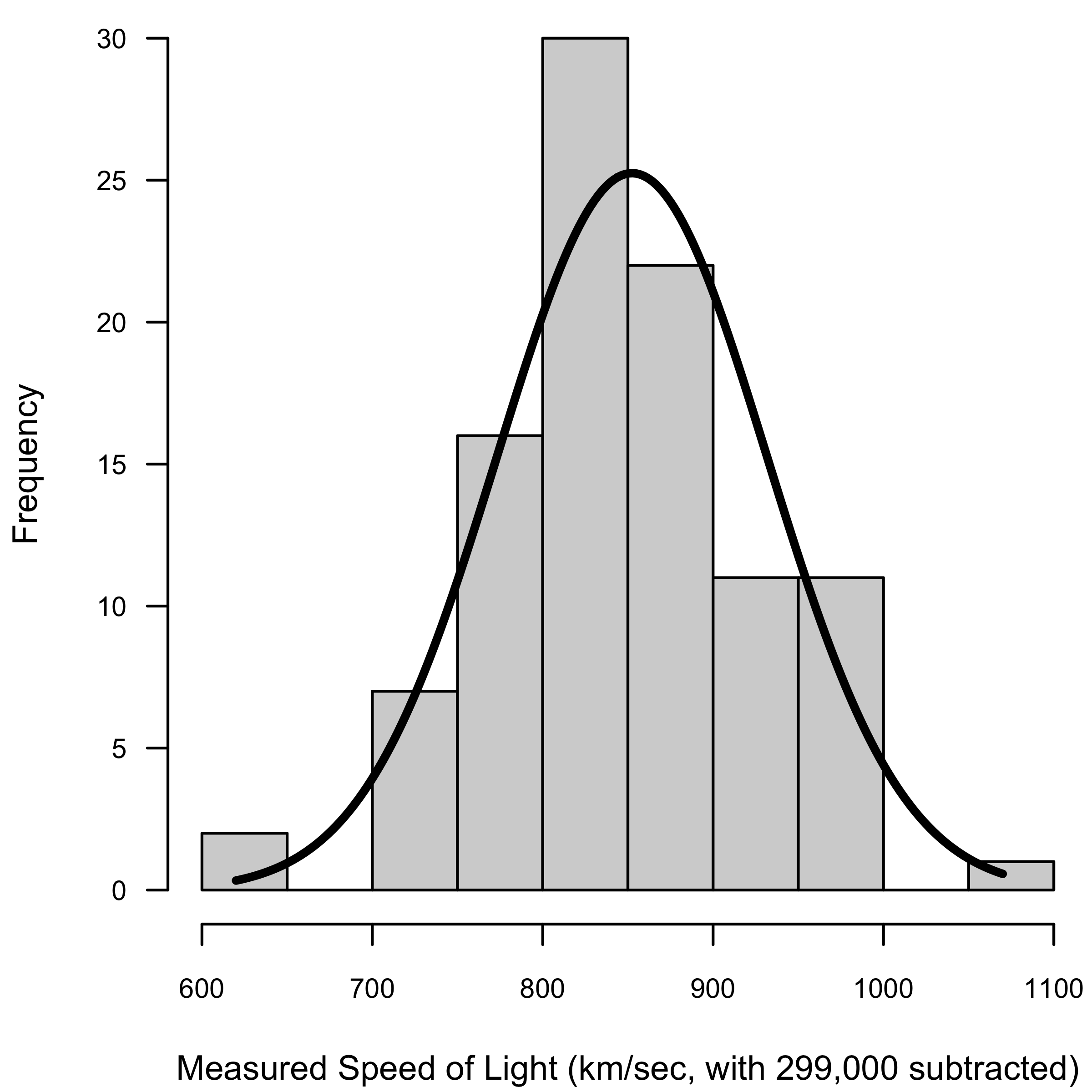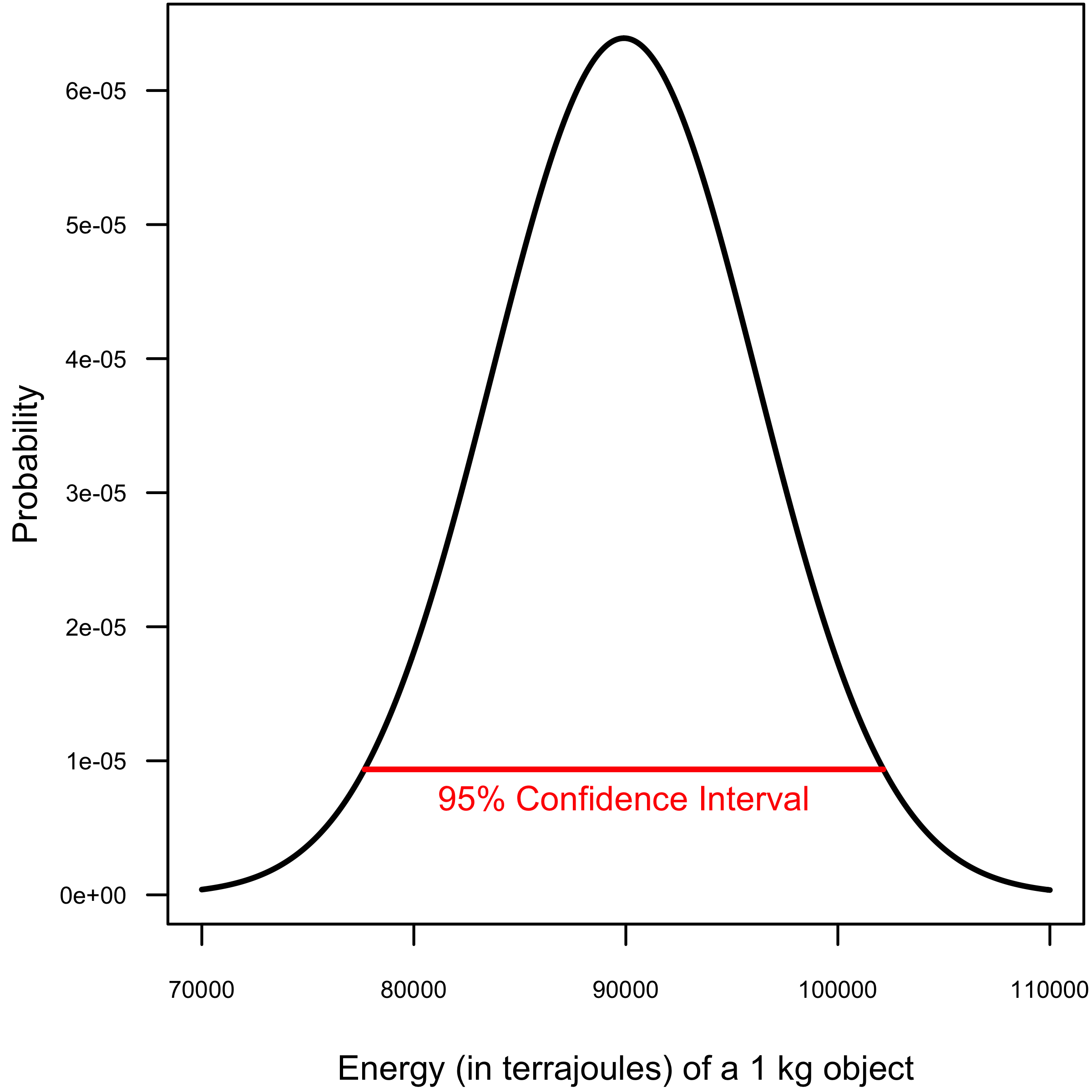# A Statistical Reformulation of the Mass-Energy Equivalence Equation, E=mc2Introduction
The mass-energy equivalence equation, E = mc2 (where E is energy, m is mass, and c is the speed of light), states that the mass of an object is proportional to its energy content. It is arguably the most famous mathematical equation in all of science, at least for non-statisticians. This equation has unified two seemingly disparate physical concepts (energy and mass), gave birth to the atomic bomb (Jha 2014), and spurred the development of nuclear technologies such as PET scans, smoke detectors, and radiocarbon dating (Tyson 2005). We also believe the equation to be slightly inaccurate, from a statistical point of view.

Scientists have long operated under the assumption that c, the speed of light, is a constant. More specifically, c is assumed to equal 299,792.458 km/s (or about 671 million miles per hour). However, experimental data has shown that this assumption of constancy is not necessarily valid. For instance, the speed of light decreases when travelling through transparent substances like water or glass (quantified by the refractive index). Additionally, virtual particles present in vacuum may cause fluctuations in the speed of light (Urban et al. 2013), although we are 95% confident that we do not know what this means.

Because the speed of light has been shown to be variable, it should not be included as a constant in a deterministic mathematical model. When a variable does demonstrate stochasticity, statistical models are often preferred. Thus, in this paper, we present a statistical reformulation of the mass-energy equivalence model.

The Model
As stated above, the deterministic mass-energy equivalence model is written as:

E = mc2.     (1)

As also stated above, the speed of light, c, should be modeled as a stochastic variable, which are usually described by using a distribution (such as Poisson, Normal, Binomial, etc.). Thus, the correct form of the mass-energy equivalence equation is:

E = mc2

c ~ P,          (2)

where P represents some unknown distribution. All we have to do now is to figure out which distribution best characterizes our measurements of the speed of light.

Distribution of c
As statisticians, we are well-versed in using the R statistical platform. Thus, we are familiar with all of the example datasets that come with the basic R package. One such dataset just happens to be the measurements of the speed of light by A. A. Michelson in 1879 (Link to data, Link to paper). This dataset provides 100 speed of light measurements conducted over 20 trials in 5 different experiments. To use an adequate sample size, we will treat all 100 observations as independent. One hundred observations may seem a small amount; however, as statisticians, we also have access to the Law of Large Numbers, which lets us use a sample of any size.

To figure out which distribution to use, we first made a histogram of all 100 observations (Figure 1). From Figure 1, you can see that the histogram strongly suggests a Normal (or Gaussian) distribution. And, indeed, a Shapiro-Wilk’s test demonstrates that the distribution of the data do not significantly differ from a Normal distribution (W = 0.981, P = 0.514).Figure 1. Michelson’s data on the speed of light as a histogram, and a normal distribution fitted to this data as the solid black line

Thus, the absolute correct statistical equation for mass-energy equivalence is:

E = mc2

c ~ N(μ,σ2),        (3)

where µ is the mean speed of light and σ2 is the variance of the speed of light.

The last step is to obtain estimates of the two parameters of the Normal distribution—the mean and variance. The mean of Michelson’s data on the speed of light is 299,852.4 km/s and the variance is 6242.667 km2/s.

Application of Equation
Using the ‘old’ mass-energy equivalence equation (i.e., Eq. 1), a one-kilogram object would have 89,875.52 terrajoules of energy. Using the correct equation (i.e., Eq. 3) and the observed calculations of mean and variance from Michelson’s experiment, a one-kilogram object would contain (mean ± 1 SD) 89,911.47 ± 6,242.67 terrajoules of energy (see Figure 2). Additionally, we are 95% confident that the mean energy content of a 1 kg object is between 77,675.84 and 102,147.10 terrajoules.Figure 2. Normal distribution showing the amount of energy contained within a 1kg object. Red line shows 95% Confidence Interval

Discussion
Just like everything else in the world, the speed of light ought to be variable. And, just like everything else in the world, it ought to be modeled using a statistical equation. We have successfully updated the mass-energy equivalence model to reflect the natural variability in the speed of light.

There are probably many implications of a revised mass-energy equivalence model to different scientific fields, such as physics and quantum mechanics. There are also likely to be implications to the various applications of the mass-energy equivalence equation, such as smoke detectors and nuclear power. As statisticians, we cannot speak to these implications, although we expect to be credited and compensated accordingly.

Instead, we wish to discuss some implications that rarely get considered in scientific papers: the effects of our discovery on pop culture. As we stated in the Introduction, E = mc2 is likely the most famous equation in the world and, as such, has been represented numerous times in pop culture. Due to our statistical revision, these depictions of E = mc2 should be altered. For example, The Theory of Relativity, a sculpture on the Walk of Ideas in Berlin should be changed to include our addition (specifically, the c ~ N(μ,σ2) portion). Crew members of the USS Enterprise should reunite, spell out our addition to the equation and take a replacement photo. And, most importantly, this episode of Animaniacs should be re-written and incorporate CNUOA somehow into the ACME song. And, we should be depicted along with Albert Einstein in the cartoon. They’re writers, they can figure it out.

Conclusion
With our revised equation, we have successfully unified the physical concepts of energy and mass with the mathematical concept of statistics. We are fully aware that further unification is possible. For example, Einstein unified the mass-energy equivalence with space and time to create the General Theory of Relativity. We have now paved the way for a Statistical General Theory of Relativity. It has not escaped our attention that if a Bayesian Statistical General Theory of Relativity is developed, it will likely become the new Grand Unified Theory in physics.